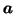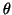Next: Stabilising the Fixed-Point Update Up: Update Rules Previous: Update Rules

### Avoiding Problems Originating from Approximation of the Nonlinearity of the Hidden Neurons.

The approximations in (24) and (25) can give rise to problems with ill defined posterior variances of sources or first layer weights A or biases. This is because the approximations take into account only local behaviour of the nonlinearities g of the hidden neurons. With MLP networks the posterior is typically multimodal and therefore, in a valley between two maxima, it is possible that the second order derivative of the logarithm of the posterior w.r.t. a parameteris positive. This means that the derivative of the Cp part of the cost function with respect to the posterior varianceof that parameter is negative, leading to a negative estimate of variance in (28).

It is easy to see that the problem is due to the local estimate of gsince the logarithm of the posterior eventually has to go to negative infinity. The derivative of the Cp term w.r.t. the posterior variancewill thus be positive for large, but the local estimate of g fails to account for this.

In order to discourage the network from adapting itself to areas of parameter space where the problems might occur and to deal with the problem if it nevertheless occurred, the terms in (24) which give rise to negative derivative of Cp with respect towill be neglected in the computation of the gradients. As this can only make the estimate ofin (28) smaller, this leads, in general, to increasing the accuracy of the approximations in (24) and (25).Next: Stabilising the Fixed-Point Update Up: Update Rules Previous: Update Rules
Harri Lappalainen
2000-03-03